# Javascript parseFloat: Examples and variations of this function

Javascript is a scripting language and it comes with a vast amount of methods and in-built functions. We can use these methods and in-built functions in various ways with different data types. Various in-built functions are associated with the string data type in javascript. One of the most useful of them is the javascript parseFloat method.

We can use the parseFloat method to convert a string into a floating-point number. The parseFloat method takes a string value and returns a floating-point number. We can use the parseFloat method in various ways in string manipulation. But there are few rules for using parseFloat method in javascript. We will discuss these rules of using parseFloat method in this article.

### Examples of parseFloat in javascript

Let’s understand the parseFloat method in javascript with the help of a simple example.

#### Example 1

`var result = parseFloat("100");`

The output is: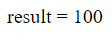In the above example, we used the parseFloat method and passed a string value. The string value equals 100. The parseFloat methods an integer value that equals 100. This is how the parseFloat method works in java. A string value containing numbers is passed and a corresponding integer is returned. But the parseFloat method only converts the first number of a string into an integer. Let’s understand this with the help of an example.

#### Example 2

`var result = parseFloat("10 20 30");`

The output is: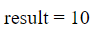We pass the string value of “10 20 30” in the parseFloat method. But the value returned by the parseFloat method is only 10. This is because the first number in the string we pass is 10. After 10, there is whitespace. The parseFloat method does not count anything after whitespaces in the string. In fact, it does not count anything in the string after any non-number character. Observe the following example.

#### Example 3

`var result = parseFloat("This is 10");`

The output is: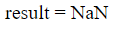We passed “This is 10” in the parseFloat function. Even there is a number in the string we passed but the output is NaN(Not a Number). This happens because the number in the string is after the alphabets. This is not allowed in parseFloat methods. But trailing and leading whitespaces are allowed in the parseFloat method. The same happens when a number in string is in front of non-number characters.

#### Example 4

`var result = parseFloat("10 is a number");`

The output is:#### Example 5

`var result = parseFloat("    100    ");`

The output is:We passed a string containing a number in the parseFloat method. But the string also contains trailing and leading whitespaces. Even because of the whitespaces, the value returned is 100. This is because the trailing and leading whitespaces are allowed in the parseFloat method.

We have to remember that if the first character of the string passed in the parseFloat method is not a number, the result will be NaN.

### Using parseFloat with decimals

The parseFloat method in javascript can also be used with decimals. We can pass a string containing a decimal number and get the corresponding floating-point number. The following example shows a decimal number passed in a parseFloat method.

` var result = parseFloat("100.33445");`

The output is: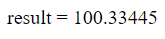In the above code, we pass “100.33445” to the parseFloat method. The returned value is exactly the same. This is how the parseFloat method works with decimals.

We can also fix the numbers after decimal the help of toFixed methods. We have to pass a number in the toFixed method and the resulting integer will have that many numbers of digits after the decimal. The following example shows how to use the toFixed method with the parseFloat.

``` var result = parseFloat("100.334445").toFixed(2);
```

The output is: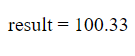In the above code, we use a toFixed method and passed a value of 2 in it. The string we passed in the parseFloat method has five digits after the decimal but the returned value has only two. This is because of the toFixed method. The value returned is always a round-off value. But what happens when no value is passed in the toFixed method?

`var result = parseFloat("100.334445").toFixed();`

The output is:The value returned has no decimal when there no value passed in the toFixed method.

### Using parseFloat with commas

Like other non-numbers, no character will be considered after a comma in the string passed in the parseFloat method. The only number in the string will be returned by the parseFloat method. The following is an example of parseFloat with a comma.

`var result = parseFloat("10,12")`

The output is: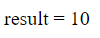In the above code, we passed a string with a comma in the parseFloat method. The output is only the number before a comma.

### Conclusion

Javascript is a scripting language that provides many in-built functions that are very helpful while coding. The parseFloat is an in-built function used to parse any numbers in a string to a floating-point method. It is immensely useful while tracking numbers in a string. But there are few rules of using the parseFloat function that we discussed in this article.

0Shares